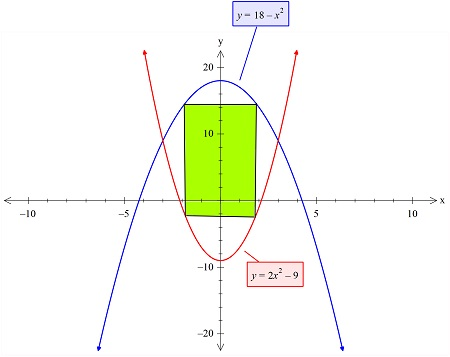# Find the area of the largest rectangle (with sides parallel to the coordinate axes) that can be...

## Question:

Find the area of the largest rectangle (with sides parallel to the coordinate axes) that can be inscribed in the region enclosed by the graphs of f(x) = 18 - x{eq}^2 {/eq} and g(x) = 2x{eq}^2 {/eq} - 9.

## Finding Minima & Maxima:

We need to find the area of a rectangle that is inscribed between two curves. To do this, we'll use the concept of differentiation and the technique of solving a linear equation in one variable.

We have plotted the two graphs and the rectangle (in green).The area of this rectangle is given as:

{eq}A'(x)= 2x* 2y\\ = 4 xy \\ =4 x(18-x^2-(2x^2-9)) \\ =4x\left(-3x^2+27\right)\\ {/eq}

This area needs to be maximized, so we will use differentiation as follows:

{eq}A'(x)=0\\ \Rightarrow \frac{d}{dx}\left(4x\left(-3x^2+27\right)\right)=0\\ \Rightarrow 4\left(-9x^2+27\right)=0\\ \Rightarrow x =\sqrt{3}\\ {/eq}

So at this value of x, the area of the rectangle is maximum.